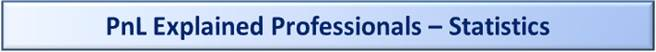Statistics Main Page

Overview

Resources related to Statistics.  Some items are from my Introduction to Statistics class from NYU (New York University, Stern School of Business).

Probability and Statistics in 10 Minutes

Click on these links for and intro to concepts, terminology and symbols for Probability and Statistics.

Part 2: Probability in 10 Minutes

Sample Midterms and Finals

See here for Sample Midterms and Finals, one version with Questions only and another with both the Questions and the Answers, i.e., the Answer Key

Probability Versus Statistics

The full name of the topic is ‘Probability and Statistics’, which is often condensed to just the term ‘Statistics’, though they are technical two separate things:

1) ‘Probability’ as a topic relates to calculating the odds of something.  E.g. if you roll a pair of dice, what are the odds you get a 7.   The odds for that are 6/36, by the way.  Interpreted as 6 out of every 36 rolls will yield a pair of dice that sum to 7.  That could be a 1 plus a 6, a 2 plus a 5 or a 3 plus a 4 or vice-versa.

2) ‘Statistics’ relates to these two concepts:

2.1) Calculating a ‘Statistic’, which is a descriptor of a certain set of numbers.  The most common statistics are

2.1.1) The ‘Average’, a.k.a., ‘Arithmetic Mean’ and

2.1.2) The ‘Standard Deviation’, which is the square root of the ‘Variance’.

If numbers are like nouns, then Statistics are like adjectives, the words that describe them.

For example, if you have the heights of 10 people in a room, you might describe that set of numbers by giving the average.  You might say, the average height is 5 feet and 6 inches.  Or 1.67 meters, for my friends currently enjoying the metric system.

2.2) The second part relates to Statistical Testing.  This includes topics such as sampling, sample design, and estimating values.

2.2.1) Point Estimates, Confidence Intervals, and Confidence Levels

Estimating a value would typically have both your ‘point estimate’, i.e., one number that is your best guess, I mean, best estimate based on the data, and then, typically, also a range, which is called ‘Confidence Interval’.

A confidence interval is two sided, e.g., like they’ll say, typically, a given election poll has a certain Point Estimate, plus or minus 3%  The plus or minus part describes the Range that is the ‘Confidence Interval’.

A ‘Confidence Level’ is one-sided.  This is the case where you are concerned only with items being above.. or, alternately, being below a certain threshold.  An example of this from Finance is the concept in Market Risk called ‘VaR’ or ‘Value-at-Risk’.  A VaR calculation is typically concerned with losses with less than a 5% threshold, i.e., ‘confidence level’.  Or, alternately, a 1% level.

An Unbiased Estimator of the Variance

If you have ever taken an Intro to Statistics class, see if this has happened to:

Your teacher tells you to use ‘n – 1’ instead of ‘n’ when calculating the Standard Deviation or Variation of a set of numbers.  Where ‘n’ stands for ‘number of items in the sample’, e.g., if your sample size was 10, then ‘n – 1’ would be 9.

This is confusing for two reasons:

1) Your teacher never explains it.  You get a ‘just do it this way’ response.  Translation?  Your teacher likely doesn’t know.

2) By comparison, when you are told that when you calculate the sample mean, you just use the sample size, i.e., the ‘n’ and not the ‘n – 1’.  You’re smart, so you recognize the inconstancy.

For those of you familiar with Excel, these are the functions involved:

For Standard Deviation:

STDEVP for the calc that uses ‘N’.

STDEV for the calc that uses ‘N - 1’.

For Variance:

VARP for the calc that uses ‘N’.

VAR for the calc that uses ‘N - 1’.

Note:  don’t confuse ‘VAR’ here, which means ‘Variance’, with the acronym ‘VaR’, which is mentioned above as an example of statistics, which means ‘Value-at-Risk’.

In the Excel functions, the ‘P’ at the end is for ‘Population’, which means the complete set of all possible values of a distribution.   The version without the ‘P’ is used for a ‘Sample’.

What is provided here is the best and most intuitive description you’ll ever see explaining why the right answer for an unbiased estimator of the variance or standard deviation has to be the ‘N – 1’ version.